# Writing an equation and drawing its graph to model a real-world situation: Basic Online Quiz

Following quiz provides Multiple Choice Questions (MCQs) related to Writing an equation and drawing its graph to model a real-world situation: Basic. You will have to read all the given answers and click over the correct answer. If you are not sure about the answer then you can check the answer using Show Answer button. You can use Next Quiz button to check new set of questions in the quiz.Q 1 - Stacy is putting $250 in a savings account and adding$40 each week. Let y represent the total amount saved and let x represent the number of weeks Stacy has been adding money. Write an equation relating y and x and graph it.

### Explanation

Step 1:

The equation representing the problem in x and y is y = 250 + 40x

Step 2:

The plot is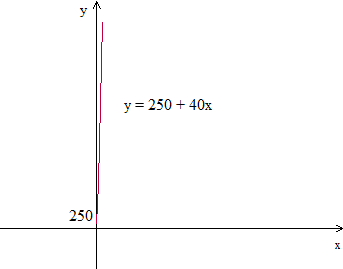Q 2 - Jenny has savings of $42 and earns$7 for each hour of lawn mowing. If y is the total amount with Jenny and x is the number of hours he works, write and equation in y and x and graph it.

### Explanation

Step 1:

The equation representing the problem in x and y is y = 42 + 7x

Step 2:

The plot is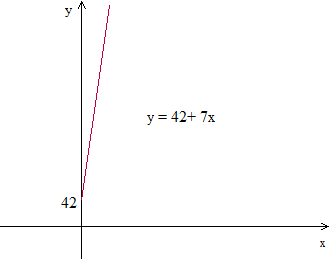Q 3 - Stella has walked 18 kilometers, further she plans to walk 2 kilometer during each trip to work. Let y be the total distance walked and x be the number of trips she makes. Write an equation an equation in x and y and graph it.

### Explanation

Step 1:

The equation representing the problem in x and y is y = 18 + 2x

Step 2:

The plot is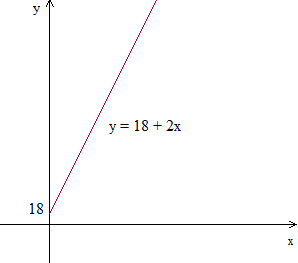Q 4 - Punto Car Rentals charge a base charge of $75 and$21 per hour for renting cars. Let y represent the total bill amount and x be number of hours the car is rented. Write an equation relating y and x and graph it.

### Explanation

Step 1:

The equation representing the problem in x and y is y = 75 + 21x

Step 2:

The plot is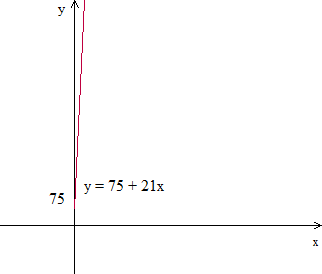### Explanation

Step 1:

The equation representing the problem in x and y is y = 110 + 7x

Step 2:

The plot is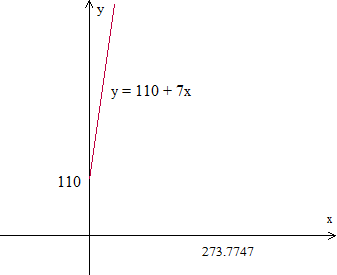Q 8 - Nora has made 15 liters of jam and will make an additional 2 liter of jam every day. If y is the number of liters of jam made and x is the number of days, write an equation relating y and x and graph it.

### Explanation

Step 1:

The equation representing the problem in x and y is y = 15 + 2x

Step 2:

The plot is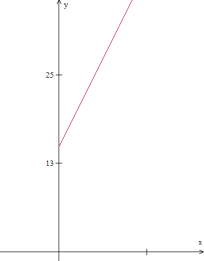Q 9 - Keira has already written 35 pages and she writes 6 pages per hour. Let y be the total pages written and x the number of hours she writes. Write an equation in y and x and graph it.

### Explanation

Step 1:

The equation representing the problem in x and y is y = 35 + 6x

Step 2:

The plot is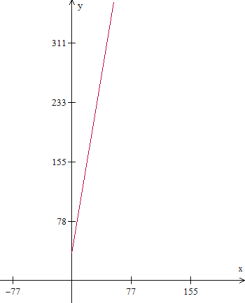Q 10 - Curtis has 6 stamps and buys 2 stamps during each day of vacation. Let y be the total number of stamps Curtis has and x be the days of vacation. Write an equation in x and y and graph it.

### Explanation

Step 1:

The equation representing the problem in x and y is y = 6 + 2x

Step 2:

The plot is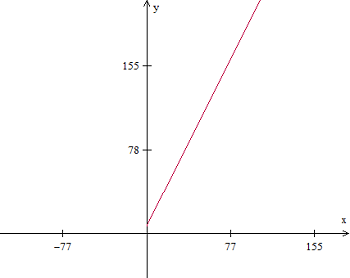writing_an_equation_and_drawing_its_graph_to_model_real_world_situation_basic.htm